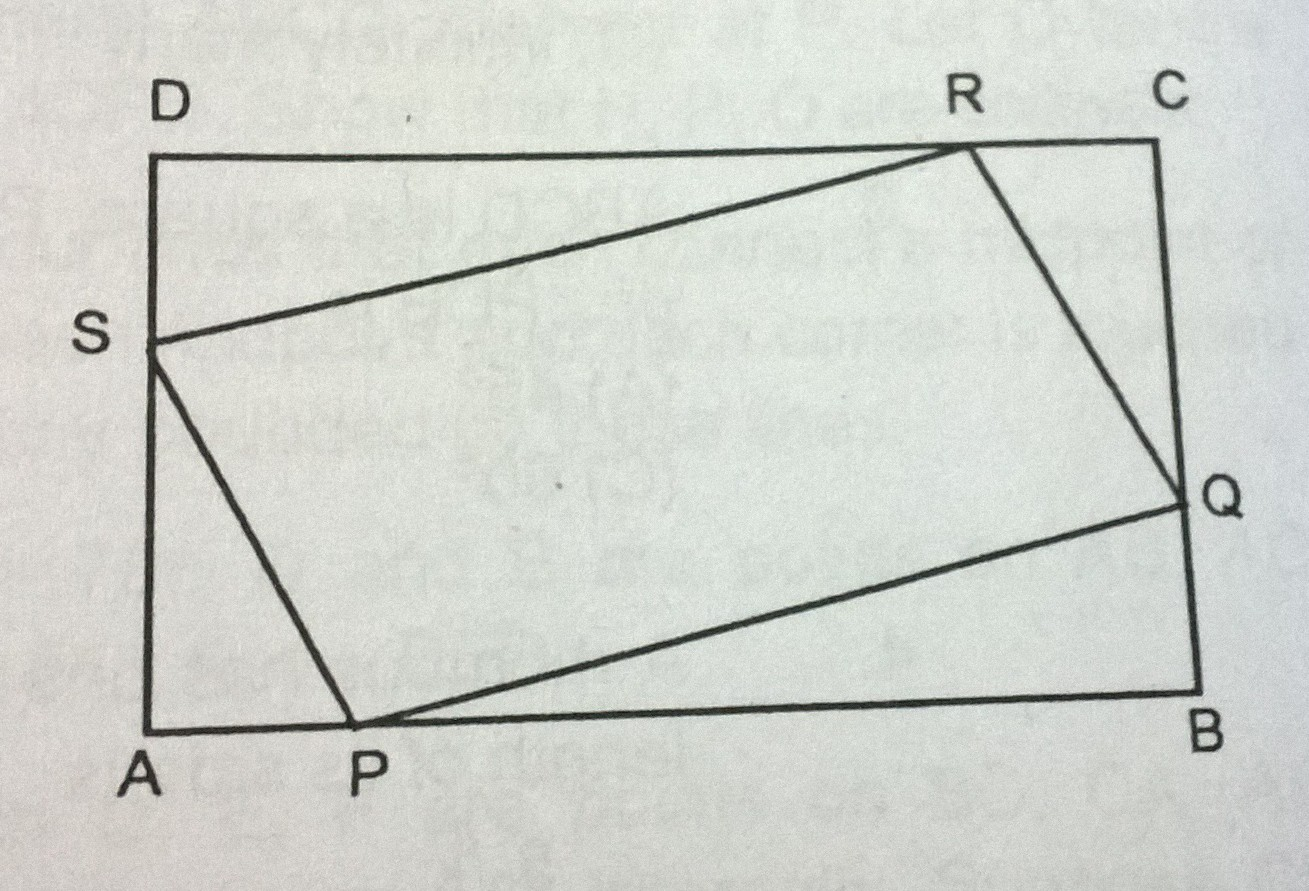# easy geo-metry...

Geometry Level 2Points P , Q , R and S divide the sides of a rectangle ABCD in the ratio 1 : 2 as shown in the figure. What fraction of area of the rectangle is the area of parallelogram PQRS ?

×